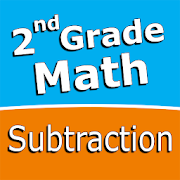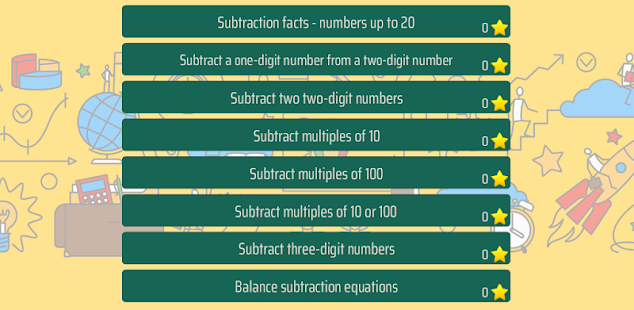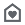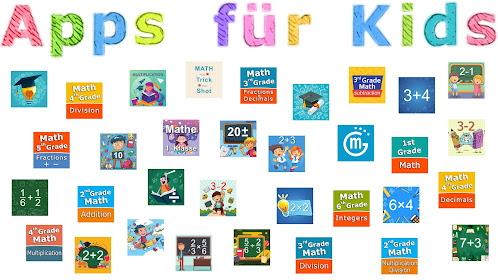# Second grade Math - SubtractionEveryone
7Intuitive interface powered by handwriting input and a fun and engaging mini game in addition to a regular math trainer mode make our app stand out from the crowd of generic math learning apps.

With Second grade Math - Subtraction you can practice and improve the following math skills:
- Subtraction facts - numbers up to 20
- Subtract a one-digit number from a two-digit number
- Subtract two two-digit numbers
- Subtract multiples of 10
- Subtract multiples of 100
- Subtract multiples of 10 or 100
- Subtract three-digit numbers
- Balance subtraction equations
Collapse

## Reviews

Review policy and info
2.9
7 total
5
4
3
2
1

Eligible for Family LibraryEligible if bought after 7/2/2016. Learn More
Updated
June 6, 2020
Size
Varies with device
Installs
500+
Current Version
Varies with device
Requires Android
Varies with device
Content Rating
Everyone
Permissions
Offered By
Sergey Malugin